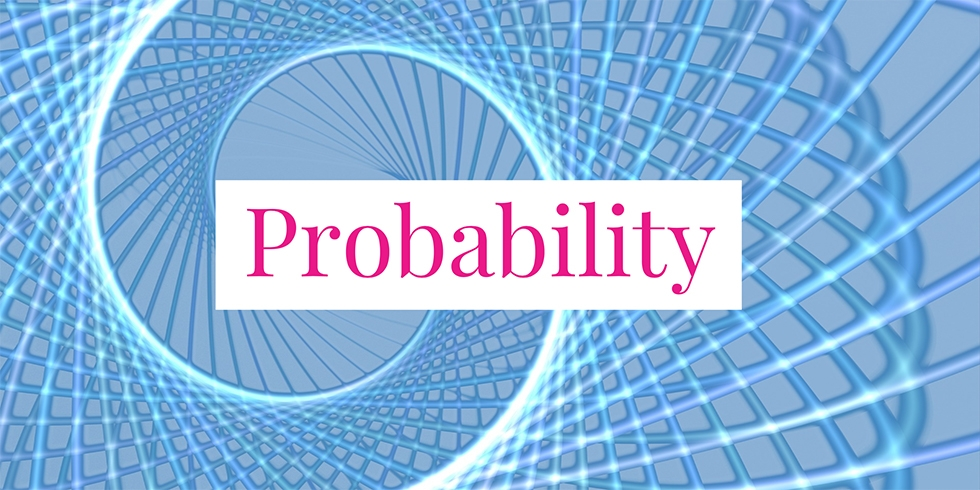# What is the Probability that Selected Coin was Two-Headed?## Problem 739

There are three coins in a box. The first coin is two-headed. The second one is a fair coin. The third one is a biased coin that comes up heads $75\%$ of the time. When one of the three coins was picked at random from the box and tossed, it landed heads.

What is the probability that the selected coin was the two-headed coin?Add to solve later

## Hint.

Use Bayes’ theorem (Bayes’ rule).

## Solution.

Let $E_i$ be the event of the $i$-th coin being picked for $i = 1, 2, 3$. Let $F$ be the event that a coin lands heads.

The required probability can be calculated using Bayes’ rule as follows:
$P(E_1 \mid F) = \frac{P(F \mid E_1) \cdot P(E_1)}{P(F)}.$ When the two-headed coin is picked, it always lands heads. Thus, we have the conditional probability $P(F \mid E_1) = 1$. The probability that the two-headed coin is selected out of the box is $P(E_1)=1/3$.

The probability in the denominator is calculated using the total probability theorem as follows:
\begin{align*}
P(F) &= \sum_{i=1}^3 P(F \mid E_i) P(E_i) \6pt] &= \frac{1}{3}\left(1+\frac{1}{2} + \frac{3}{4} \right)\\[6pt] &= \frac{3}{4}. \end{align*} It follows by the formula above that the required probability is \[P(E_1 \mid F) = \frac{1\cdot \frac{1}{3}}{\frac{3}{4}} = \frac{4}{9}.Add to solve later

### More from my site

#### You may also like...

This site uses Akismet to reduce spam. Learn how your comment data is processed.

###### More in Probability##### If a Smartphone is Defective, Which Factory Made It?

A certain model of smartphone is manufactured by three factories A, B, and C. Factories A, B, and C produce...

Close